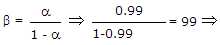# Electronics and Communication Engineering - Electronic Devices and Circuits

1.
At room temperature the current in an intrinsic semiconductor is due to
holes
electrons
ions
holes and electrons
Explanation:

Intrinsic material has equal number of holes and electrons.

2.
Work function is the maximum energy required by the fastest electron at 0 K to escape from the metal surface.
True
False
Explanation:

Work function is the minimum energy required by the fastest electron at 0 K to escape from the metal surface.

3.
The most commonly used semiconductor material is
silicon
germanium
mixture of silicon and germanium
none of the above
Explanation:

Germanium is rarely used.

4.
In which of these is reverse recovery time nearly zero?
Zener diode
Tunnel diode
Schottky diode
PIN diode
Explanation:

In schottky diode there is no charge storage and hence almost zero reverse recovery time.

5.
A transistor has a current gain of 0.99 in the CB mode. Its current gain in the CC mode is
100
99
1.01
0.99Current gain = 1 + β = 100.